# Uncountability: Intuitive Intro

Collections which have less than or the same number of items than the collection of all natural numbers are called countable, while larger collections (like the set of all real numbers) are called uncountable.

All uncountable collections (and some countable collections) are infinite and some infinite collections are larger than others noteAt least, within mathematical systems which include the Axiom of Choice, see the technical page for details.. To demonstrate this, we’ll explore a graphical demonstration with tiles and paths.

## Tiles and paths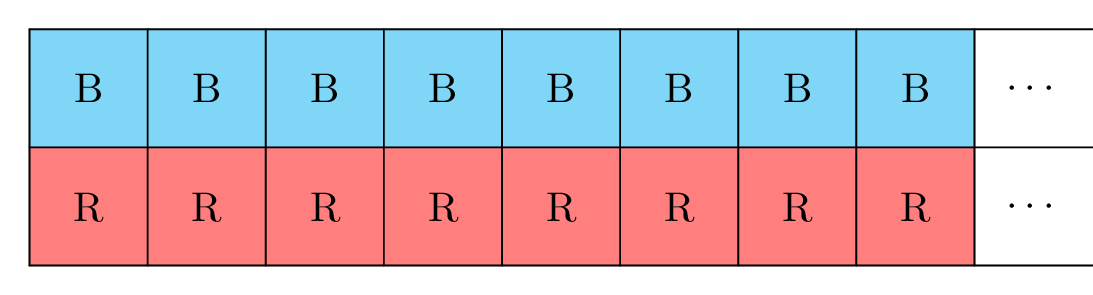Consider, as shown above, a sidewalk that goes on forever in one direction, which is made up of equal-sized square tiles. The sidewalk is two squares across. Consider a person who walks forever on it, obeying the following rule: Each step the person takes must be to one of the two tiles immediately in front of that person; no going backwards, no skipping tiles, no going sideways, no standing in place forever. The following is the beginning of one possible path: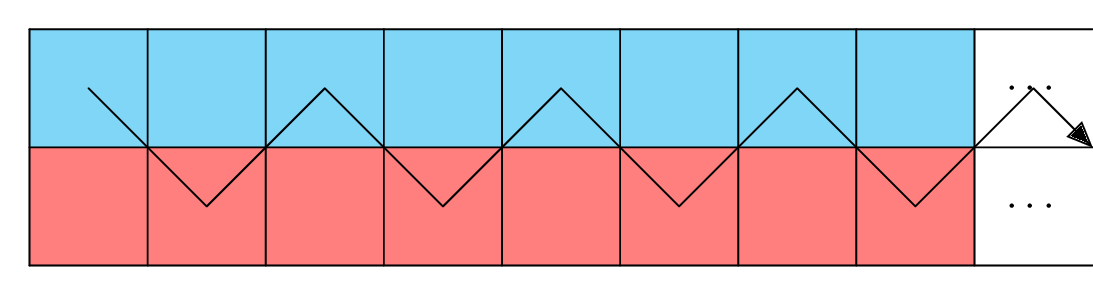1. How many tiles are there?

2. How many possible paths are there?

In both cases, you could just say that there are infinitely many, and that would be correct. But now let’s consider a third question:

1. Is the number of tiles the same as the number of possible paths?

It turns out that there is a meaningful and well-defined way to compare the sizes of different infinite collections of things, and some infinite collections are larger than others. In particular, some infinite collections are countable (like the set of all natural numbers), while others are uncountable (like the set of all real numbers). As we will see, it can be shown that the number of tiles on our infinite sidewalk is countable, but that the number of possible paths one could take, following the rules above, is uncountable. So there are in fact more possible paths than there are tiles.

Let’s dig into exactly what this means and why it’s true.

## Pairing off

We say that two collections of things are the “same size” if you can match the items together completely: you can pair each of the things in the first collection with exactly one of the things in the second collection, in such a way that there is nothing left unpaired. For example, given two sets of three things each, we may pair them. Here is an example of such a pairing: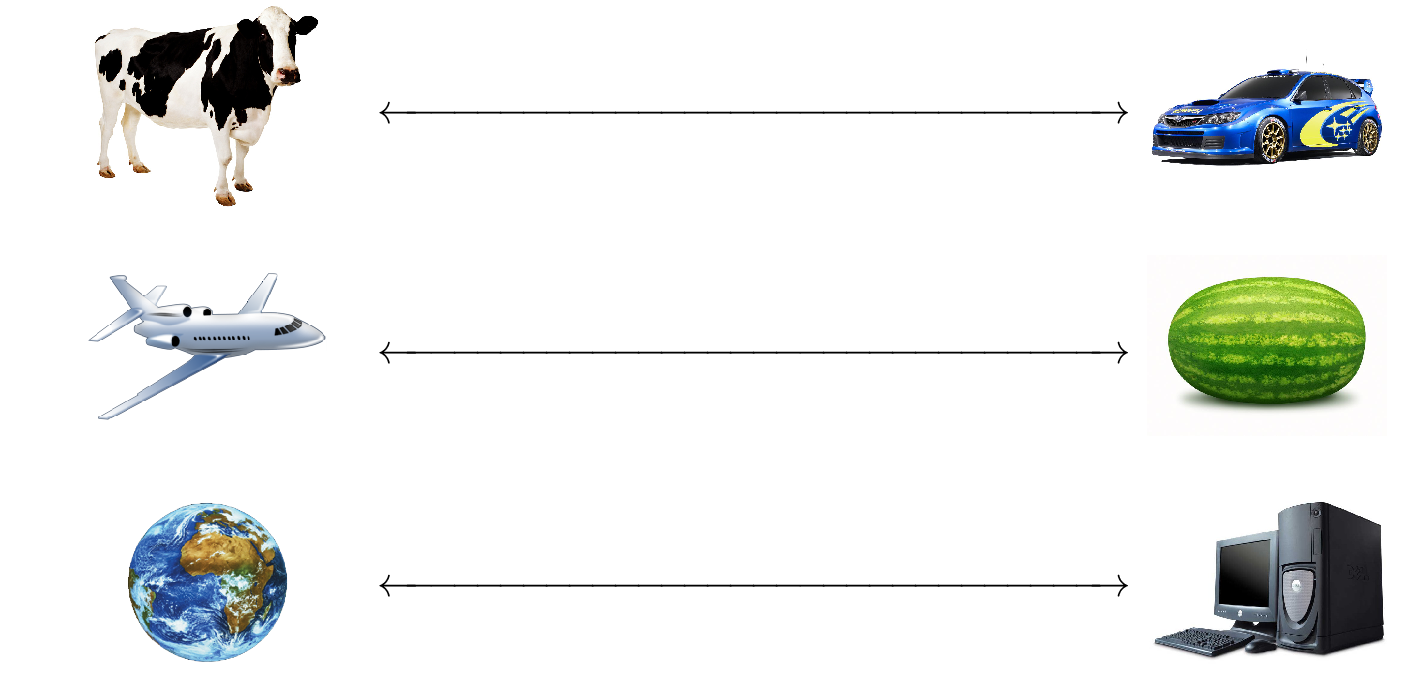You might think it obvious, then, that the number of paths our person can walk is bigger than the number of tiles. We can match each tile with the path that starts on a tile the same color as it, and changes to the other color after it hits this tile. For example, we would match the third red tile with the path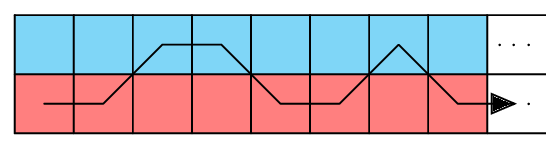It is important to note, however, that it is not sufficient that we find some matching that leaves things left over. We must show that every matching leaves things left over. For example, an infinite sidewalk that is one tile across has just as many tiles as an infinite sidewalk that is two tiles across, as we can see from the picture below by matching the 1R on top with the 1R on bottom, the 1B on top with the 1B on bottom, the 2R on top with the 2R on bottom, and so on.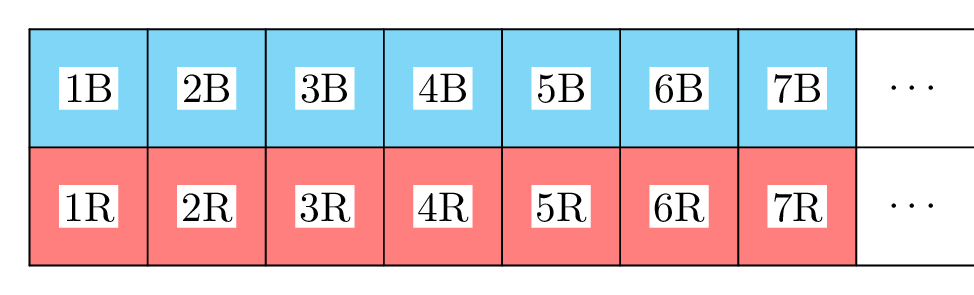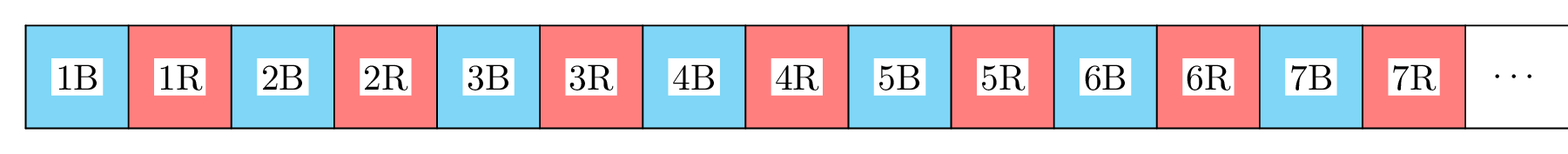In fact, if we were only to require that some matching leave extra tiles, then the number of tiles in a sidewalk that is one tile wide would not be equal to itself, for we could match the first tile with 1B (in the bottom picture above), the second tile with 2B, and so on, and we would leave over half the tiles!

In fact, even if we had a field of tiles that is infinite in every direction, we would still have no more tiles than if we had only a sidewalk that is one tile across. The following matching shows this: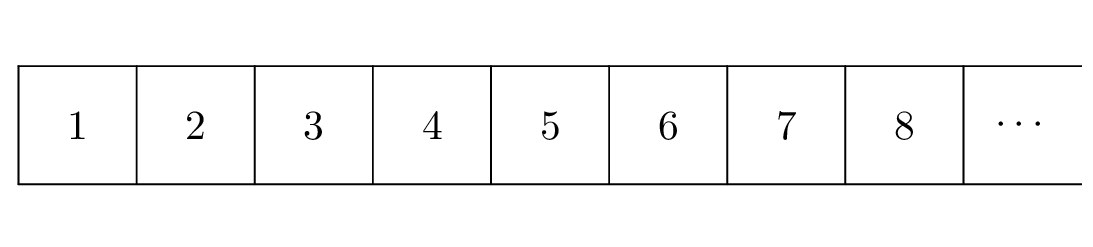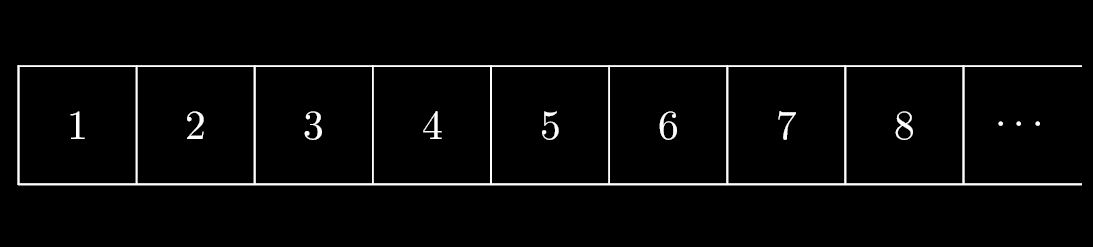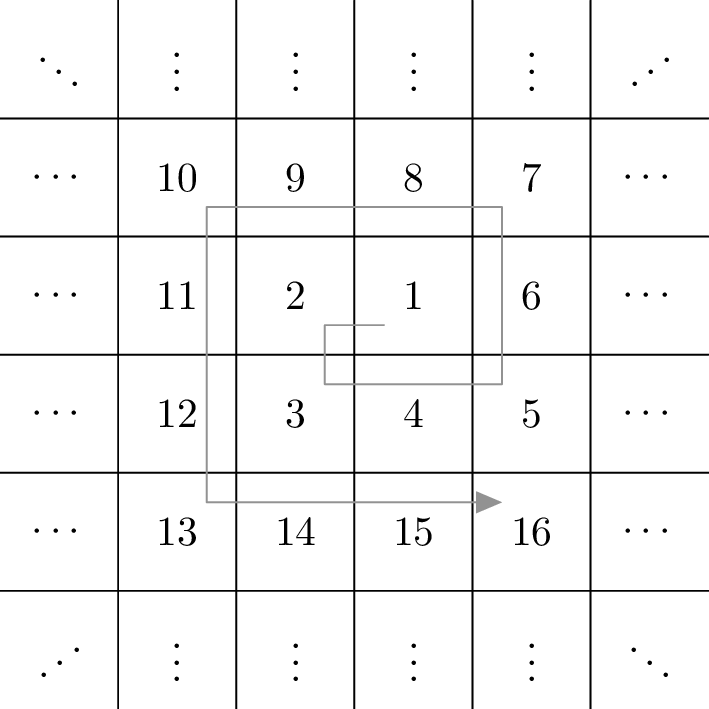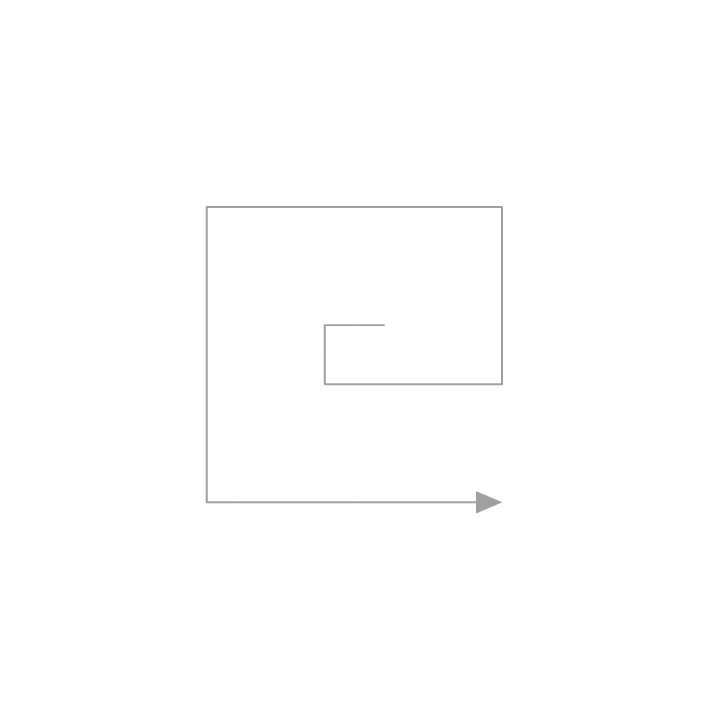## An unpairable path

You might wonder, given that there are so many different ways to match up infinitely many things, how we can know that there is no matching that catches everything. I will now prove that, no matter how you try to match paths (ways of walking) and tiles, you will miss some paths. Since we have already seen that the number of tiles in a sidewalk two tiles wide is the same as the number of tiles in a sidewalk one tile wide, I will show that any matching between paths and tiles in a sidewalk one tile wide misses some paths. I will do this by creating a path that does not match the path we have chosen for any tile noteThis type of proof is known as a proof by contradiction..

Suppose we are given a matching between tiles and paths. Since we have numbered the tiles in a sidewalk one tile wide ($\fbox{1}\fbox{2}\fbox{3}\fbox{4}\fbox{5}\fbox{6}\fbox{7}\fbox{8}\overline{\underline{\vphantom{1234567890}\cdots}}$), we also have a numbering of the paths in our matching. Consider a new path that differs from the $$n^\text{th}$$ path in our matching on the $$n^\text{th}$$ tile, that is, the $$n^\text{th}$$ step that you take. For example, if our first eight paths arethen our new path isClearly, this path is not any of the ones in the matching, because it differs from every single path at some point (in particular, it differs from the $$n^\text{th}$$ path on the $$n^\text{th}$$ tile, the $$n^\text{th}$$ step you take, which is highlighted in yellow).

Because we can repeat this exercise no matter what matching we’re given, that means any possible matching will always leave out at least one path. Thus, the number of paths a person can take must be strictly larger than the number of tiles in the sidewalk.

If you enjoyed this explanation, consider exploring some of Arbital’s other featured content!

Arbital is made by people like you, if you think you can explain a mathematical concept then consider contributing to arbital!

Parents:

• Uncountability

Some infinities are bigger than others. Uncountable infinities are larger than countable infinities.

• Nice! I’ve never seen an example like this before, and it’s actually kind of surprising. It seems to imply that 2^N is qualitatively different than N or N^2, but I’m not sure how to phrase it. (And I wonder if there is a relationship between that and P=NP problem.)

• Thanks!

A^B is the set of functions from B to A. So 2^N is powerset of N (a function f from N to {0, 1} says, for each element of N, whether or not that element is in the subset defined by f), which is isomorphic to the reals. Perhaps this should go somewhere in one or more of the versions. I don’t know any connection between this and P=NP (although I suppose it could be behind the exponential bounds on various things).

• A summary of the relevant cardinal arithmetic, by the way (in the presence of choice): $$\aleph_{\alpha} + \aleph_{\alpha} = \aleph_{\alpha} = \aleph_{\alpha} \aleph_{\alpha}$$$while $$2^{\aleph_{\alpha}} > \aleph_{\alpha}$$$

• Should be “two tile wide”, right?

• Which instance of that are you referring to (there are five)?

• Can the image below be cropped? The excessive whitespace is distracting.